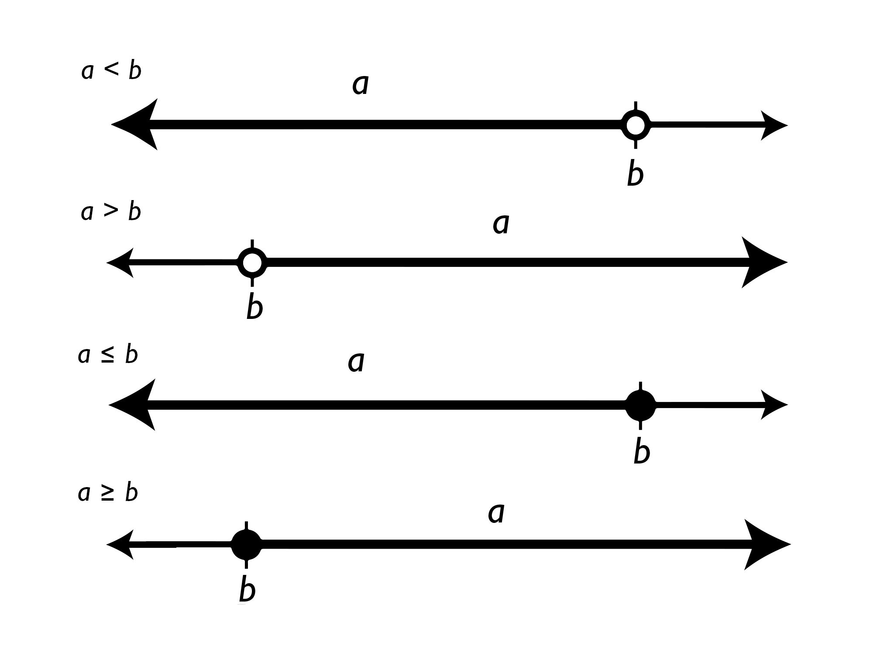# Summary of the Math: Inequalities

• An inequality is a relationship that holds between two values that are different.

• a < b means that a is less than b (or, equivalently, that b is greater than a).

• a > b means that a is greater than b (or, equivalently, that b is less than a).

• ab means that a is less than or equal to b (or, equivalently, that b is greater than or equal to a).

• ab means that a is greater than or equal to b (or, equivalently, that b is less than or equal to a).## Hint:

Can you:

• Explain the meaning of each inequality symbol?
• Show how each type of inequality is represented on a number line?